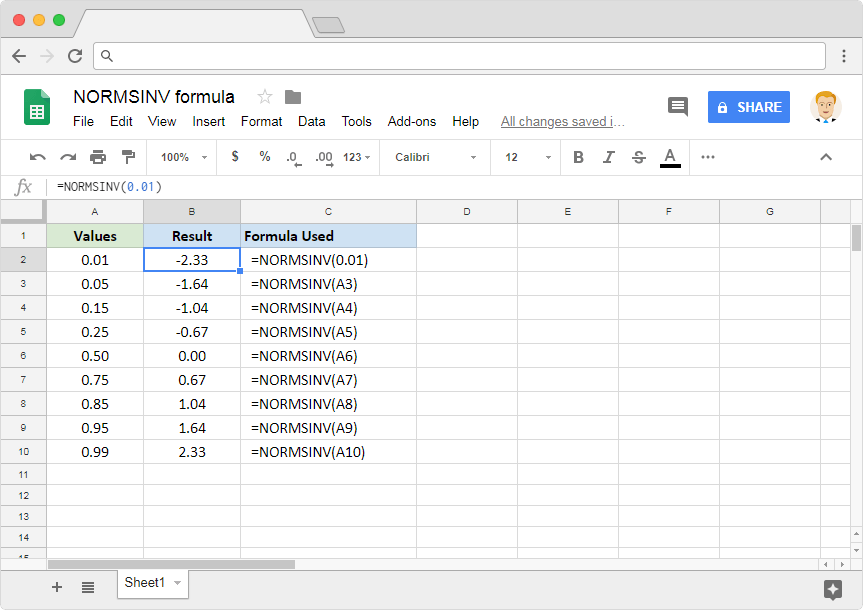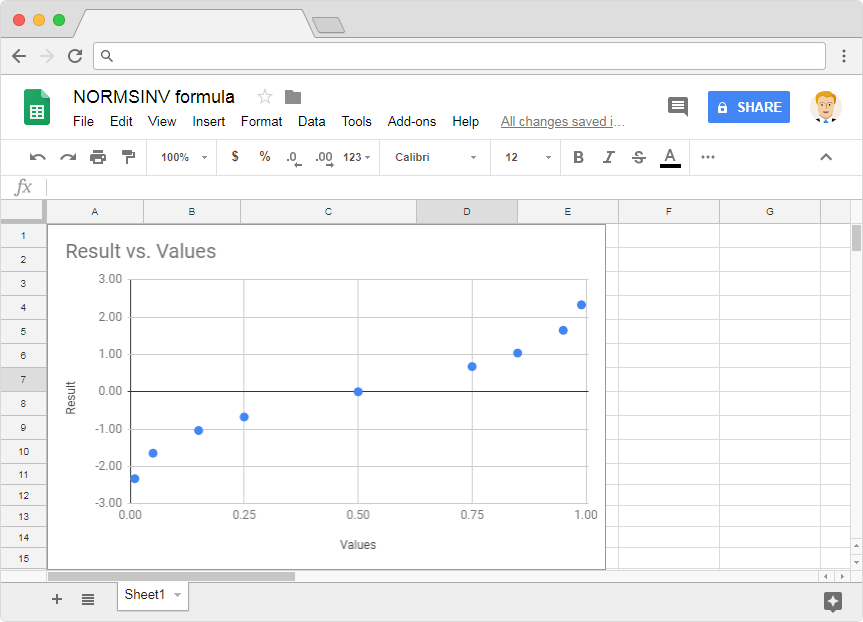# How to use the NORMSINV formula in Google SheetsWe are aware of the important role, the normal cumulative distribution plays in the field of statistics. We can derive a specialized case of normal cumulative distribution when mean is zero and the variance is 1. This is called the standard normal cumulative distribution function. In this post, we discussed how to calculate the value of this distribution function, at a specified distributed variable (x). But there is also a way to do the opposite. That is to find the value of the distributed variable, given the value of the distribution itself. The NORMSINV formula in Google Sheets helps us achieve this calculation.

### Syntax

NORMSINV(x)

• x – is the input value or cell reference that we pass to the standard normal cumulative distribution function.

Similar to the NORMSDIST formula, this also has got one of the most straightforward syntaxes in all of the Google Sheets functions. All we need to do is specify the value or cell reference of one single parameter. And it returns the value of the inverse standard normal distribution function for that specified value.

Please note that the input values should be within the confines of 0 and 1 (both not included). Otherwise, the formula returns a #NUM! error.

### Usage: NORMSINV formula in Google Sheets

While the syntax helps us to some extent, applying it practically and seeing how it behaves gives us more perspective and insights. So, let us dive right into it with a few examples created on the Google Sheets application. Please have a look at the below image.Like most other Google Sheets formulas, the NORMSINV formula also is capable of accepting direct numeric values. And also references to the cells consisting of these numeric values. The first example has an input that is a direct numeric value. Whereas all other examples have cell references for its input.

Now let’s observe the output values the formula returned. If we can quickly make a chart out of these input and output values, here’s how it looks like.If we compared the standard normal cumulative distribution function with the graph that we just created, it certainly looks like an inverse!